Polarization MCQ

# Polarization MCQ

Test Description

## 10 Questions MCQ Test Topic wise Tests for IIT JAM Physics | Polarization MCQ

Polarization MCQ for IIT JAM 2023 is part of Topic wise Tests for IIT JAM Physics preparation. The Polarization MCQ questions and answers have been prepared according to the IIT JAM exam syllabus.The Polarization MCQ MCQs are made for IIT JAM 2023 Exam. Find important definitions, questions, notes, meanings, examples, exercises, MCQs and online tests for Polarization MCQ below.
Solutions of Polarization MCQ questions in English are available as part of our Topic wise Tests for IIT JAM Physics for IIT JAM & Polarization MCQ solutions in Hindi for Topic wise Tests for IIT JAM Physics course. Download more important topics, notes, lectures and mock test series for IIT JAM Exam by signing up for free. Attempt Polarization MCQ | 10 questions in 30 minutes | Mock test for IIT JAM preparation | Free important questions MCQ to study Topic wise Tests for IIT JAM Physics for IIT JAM Exam | Download free PDF with solutions
 1 Crore+ students have signed up on EduRev. Have you?
Polarization MCQ - Question 1

### When ordinary light is passed through rotating crystal, there is no variation in the intensity of the emergent light, this implies that

Detailed Solution for Polarization MCQ - Question 1

The correct answer is: Ordinary light is symmetrical about its direction of propagation

Polarization MCQ - Question 2

### Find the thickness of a quater-wave plate when the wavelength of light is equal to 5890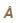and μo = 1.55 μe = 1.54. The thickness of a quater-wave plate for which μo > μe (such as calcite) is given by

Detailed Solution for Polarization MCQ - Question 2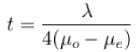Putting the given values, we get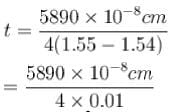= 1.47 x10-3 cm
The correct answer is: 1.47 x10-3 cm

Polarization MCQ - Question 3

### For calcite, nO =  1.65836, ne = 1.48641 at  18°C and for λ = 5893. The thickness of the quarter wave plate is :

Detailed Solution for Polarization MCQ - Question 3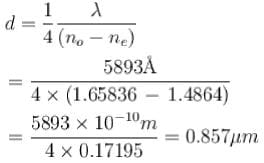The correct answer is: 0.857 μm

Polarization MCQ - Question 4

Calculate the velocities of extraordinary rays in calcite crystal in a plane perpendicular to the optic axis. Given μO = 1.658, μ= 1.486 and c = 3 x 1010 cm/s.

Detailed Solution for Polarization MCQ - Question 4

The velocity of the O-wave in the crystal is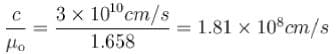= 1.81 x 108  m/s
The velocity of the E-wave in the crystal normal to be optic axis is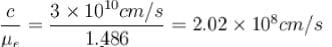= 2.02 x 10m/s
The correct answer is: = 2.02 x 10m/s

Polarization MCQ - Question 5

If the plane wave is split & recombined on a screen after the two portions, which are polarized in the x & y directions, have travelled an optical path difference of 2π/k the observed average intensity will be proportional to :

Detailed Solution for Polarization MCQ - Question 5

The correct answer is: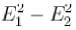Polarization MCQ - Question 6

Consider a plane wave that propagates through empty space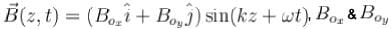are positive constants. What can you say about the polarization of this wave :

Detailed Solution for Polarization MCQ - Question 6

The correct answer is: E is always parallel to the constant vector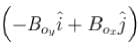Polarization MCQ - Question 7

When scattered blue light is seen through a rotating nicol prism, a variation of intensity with zero minimum intensity is seen. What does this imply?

Detailed Solution for Polarization MCQ - Question 7

The correct answer is: The scattered light is plane polarized

Polarization MCQ - Question 8

Let dw be the work done in a quasistatic reversible thermodynamic process.
Which of the following statements about dw is correct?

Detailed Solution for Polarization MCQ - Question 8

The first law of thermodynamics can be given dQ = dU + dW
here dQ and dW both are path function and dU is state function.
For making dW a perfect differential, we take dQ = 0 So dU + dW = 0 dW = –dU and dQ = 0 occur in adiabatic process, so, dW0 is a perfect differential if the process is adiabatic.

Polarization MCQ - Question 9

A calcite plate 0.0031 mm thick is cut and polished such that its optic axis is parallel to the surface. A plane polarized white light from a polariser is incident normally on the plate. Estimate the phase difference if the emergent beam for wavelengths is 7068, and μO = 1.6521 μe = 1.4811.

Detailed Solution for Polarization MCQ - Question 9

For a negative uniaxial crystal like calcite ,μO > μe. Therefore, the path difference  between O and E-rays for calcite plate of thickness t is, Δ = ( μO- μ) t and corresponding phase difference,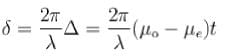Here t= 0.00031 cm
For λ = 70684,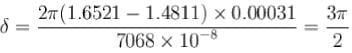As the phase difference between O and E-rays is add multiple of π/2 therefore plane polarized beam is converted into elliptically polarized light in general and circularly polarized light, if the vibration in the incident plane polarised light makes an angle of 45° with the optic axis of plate.

Polarization MCQ - Question 10

The velocity of light in water is 2.2 X 108 m/s. What is the polarizing angle of incidence?

Detailed Solution for Polarization MCQ - Question 10

Refractive index of water = Speed of light in space/Speed of light in water

= 3/2.2

= 1.3636

Using Brewster’s Law, Angle of incidence = tan-1μ

= tan-11.3636

= 53.74.

## Topic wise Tests for IIT JAM Physics

217 tests
 Use Code STAYHOME200 and get INR 200 additional OFF Use Coupon Code